SLAS525D July   2007  – December 2017

PRODUCTION DATA.

1. Features
2. Applications
3. Description
4. Revision History
5. Pin Configuration and Functions
6. Specifications
7. Detailed Description
1. 7.1 Overview
2. 7.2 Functional Block Diagram
3. 7.3 Feature Description
4. 7.4 Device Functional Modes
8. Application and Implementation
1. 8.1 Application Information
2. 8.2 Typical Applications
9. Power Supply Recommendations
10. 10Layout
1. 10.1 Layout Guidelines
2. 10.2 Layout Example
3. 10.3 Thermal Considerations
11. 11Device and Documentation Support
12. 12Mechanical, Packaging, and Orderable Information

• PFP|80
• PFP|80

## 8 Application and Implementation

NOTE

Information in the following applications sections is not part of the TI component specification, and TI does not warrant its accuracy or completeness. TI’s customers are responsible for determining suitability of components for their purposes. Customers should validate and test their design implementation to confirm system functionality.

### 8.1 Application Information

In the design of any application involving a high-speed data converter, particular attention should be paid to the design of the analog input, the clocking solution, and careful layout of the clock and analog signals. The ADS5474 evaluation module (EVM) is one practical example of the design of the analog input circuit and clocking solution, as well as a practical example of good circuit board layout practices around the ADC.

### 8.2 Typical Applications

The analog inputs of the ADS5474 must be fully differential and biased to an appropriate common mode voltage, VCM. It is rare that the end equipment will have a signal that already meets the requisite amplitude and common mode and is fully differential. Therefore, there will be a signal conditioning circuit for the analog input. If the amplitude of the input circuit is such that no gain is needed to make full use of the full-scale range of the ADC, then a transformer coupled circuit as used on the EVM may be used with good results. The transformer coupling is inherently low-noise, and inherently AC-coupled so that the signal may be biased to VCM after the transformer coupling.

If signal gain is required, or the input bandwidth is to include the spectrum all the way down to DC such that AC coupling is not possible, then an amplifier-based signal conditioning circuit would be required. Figure 43 shows LMH3401 interfaced with ADS5474. LMH3401 is configured to have to Single-Ended input with a differential outputs follow by 1st Nyquist based low pass filter with 375-MHz bandwidth. Power supply recommendations for the amplifier are also shown in the figure below.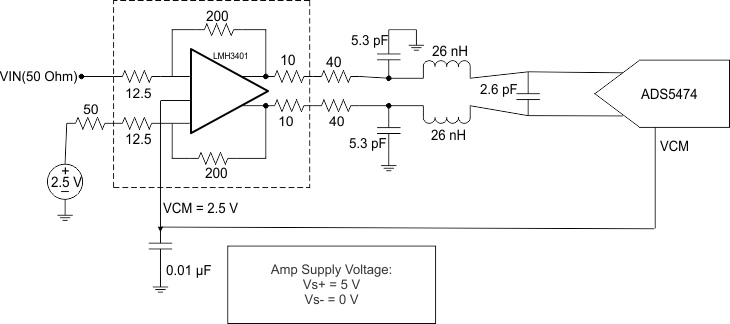Figure 43. Application Diagram

Clocking a High Speed ADC such as the ADS5474 requires a fully differential clock signal from a clean, low-jitter clock source and driven by an appropriate clock buffer, often with LVPECL or LVDS signaling levels. The sample clock is internally biased to the desired level if the sample clock is AC coupled to the ADS5474. Figure 44 shows the typical AC coupling and termination circuit used for an AC coupled clock source.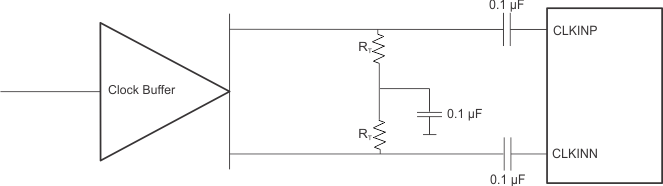Figure 44. Recommended Differential Clock Driving Circuit

#### 8.2.1 Design Requirements

The ADS5474 requires a fully differential analog input with a full-scale range not to exceed 2.2-V peak to peak differential, biased to a common mode voltage of 3.1 V. In addition the input circuit must provide proper transmission line termination (or proper load resistors in an amplifier-based solution) so the input of the impedance of the ADC analog inputs should be considered as well.

The ADS5474 is capable of a typical SNR of 70.1 dBFS for input frequencies of about 130 MHz, which is well under the Nyquist limit for this ADC operating at 400 Msps. The amplifier and clocking solution will have a direct impact on performance in terms of SNR, so the amplifier and clocking solution should be selected such that the SNR performance of at least 69 dBFS is preserved.

#### 8.2.2 Detailed Design Procedure

The ADS5474 has a max sample rate of 404 MHz and an input bandwidth of approximately 1440 MHz, but an application involving the first Nyquist zone is being considered, therefore limit the frequency bandwidth here to be under 200 MHz.

#### 8.2.2.1 Clocking Source for ADC5474

The signal to noise ratio of the ADC is limited by three different factors: the quantization noise, the thermal noise, and the total jitter of the sample clock. Quantization noise is driven by the resolution of the ADC, which is 14 bits for the ADS5474. Thermal noise is typically not noticeable in high speed pipelined converters such as the ADS5474, but may be estimated by looking at the signal to noise ratio of the ADC with very low input frequencies and using Equation 1 to solve for thermal noise. (For this estimation, we will look to the ADS5474 datasheet and take the specified SNR for the lowest frequency listed. The lowest input frequency listed for the ADS5474 is at 30 MHz, and the SNR at that frequency is 70.3 dB, so we will use 70.3 dB as our SNR limit due to thermal noise. This is just an approximation, and the lower the input frequency that has an SNR specification the better this approximation would be.) The thermal noise limits the SNR at low input frequencies while the clock jitter sets the SNR for higher input frequencies.

Quantization noise is also a limiting factor for SNR, as the theoretical maximum achievable SNR as a function of the number of bits of resolution is set by Equation 1.

Equation 1.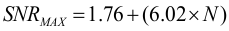where

• N = number of bits resolution

For a 14-bit ADC, the maximum SNR = 1.76 + (6.02 × 14) = 86.04 dB. This is the number that we shall enter into Equation 2 for quantization noise as we solve for total SNR for different amounts of clock jitter using Equation 2.

Equation 2.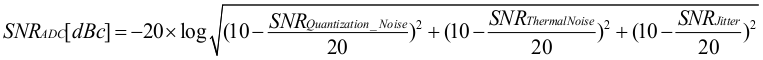The SNR limitation due to sample clock jitter can be calculated using Equation 3:

Equation 3.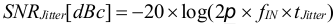The clock jitter in Equation 3 is the total amount of clock jitter, whether the jitter source is internal to the ADC itself or external due to the clocking source. The total clock jitter (tJitter) has two components – the internal aperture jitter (103 fs for ADS5474) which is set by the noise of the clock input buffer, and the external clock jitter from the clocking source and all associated buffering of the clock signal. Total clock jitter can be calculated from the aperture jitter and the external clock jitter as in Equation 4.

Equation 4.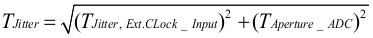External clock jitter can be minimized by using high quality clock sources and jitter cleaners as well as bandpass filters at the clock input while a faster clock slew rate may at times also improve the ADC aperture jitter slightly.

The ADS5474 has an internal aperture jitter of 103 fs, which is largely fixed. The SNR depending on the amount of external jitter for different input frequencies is shown in Figure 45. Often the design requirements will list a target SNR for a system, and Equation 1 through Equation 3 are then used to calculate the external clock jitter needed from the clocking solution to meet the system objectives.

Figure 45 shows that with an external clock jitter of 100 fs rms, the expected SNR of the ADS5474 would be greater than 69 dBFS at an input tone of 200 MHz, which is the Nyquist limit. Having less external clock jitter such as 35 fs rms or even 50 fs rms would result in an SNR that would exceed our design target, but at possibly the expense of a more costly clocking solution. Having external clock jitter of 150 fs rms or more would fail to meet the design target.

#### 8.2.2.2 Amplifier Selection

The amplifier and any input filtering will have its own SNR performance, and the SNR performance of the amplifier front end will combine with the SNR of the ADC itself to yield a system SNR that is less than that of the ADC itself. System SNR can be calculated from the SNR of the amplifier conditioning circuit and the overall ADC SNR as in Equation 5. In Equation 5, the SNR of the ADC would be the value derived from the datasheet specifications and the clocking derivation presented in the previous section.

Equation 5.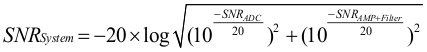The signal-to-noise ratio (SNR) of the amplifier and filter can be calculated from the noise specifications in the datasheet for the amplifier, the amplitude of the signal, and the bandwidth of the filter. The noise from the amplifier is band-limited by the filter and the rolloff of the filter will depend on the order of the filter, therefore the user should replace the filter rolloff with an equivalent brick-wall filter bandwidth. For example, a first order filter may be approximated by a brick-wall filter with bandwidth of 1.57 times the bandwidth of the first order filter. We will assume a first order filter for this design. The amplifier and filter noise can be calculated using Equation 6:

Equation 6.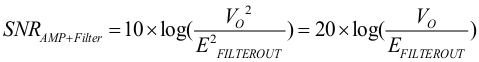where

• EFILTEROUT = ENAMPOUT × √ENB
• ENAMPOUT = the output noise density of the LMH3401 (3.4 nV / √Hz)
• ENB = the brick-wall equivalent noise bandwidth of the filter
• VO = the amplifier output signal. (which will be full scale input of the ADC expressed in rms)

In Equation 6, the parameters of the equation can be seen to be in terms of signal amplitude in the numerator and amplifier noise in the denominator, or SNR. For the numerator, use the full scale voltage specification of the ADS5474, or 2.2 V peal to peak differential. Because Equation 6 requires the signal voltage to be in rms, convert 2.2 V p-p to 0.7766 V rms.

The noise specification for the LMH3401 is listed as 3.4 (nV/√Hz), so we will use this value to integrate the noise component from DC out to the filter cutoff, using the equivalent brick wall filter of 200 MHz × 1.57, or 314 MHz. 3.4 (nV/√Hz) × 314 MHz yields 60248 nV, or 60.25 µV.

Using 0.7766 V rms for VO and 60.25 µV for Efilterout, the SNR of the amplifier and filter as given by Equation 6 is approximately 82.2 dB.

Taking the SNR of the ADC as 69.2 dB from Figure 45, and SNR of the amplifier and filter as 82.2 dB, Equation 5 predicts the system SNR to be 68.99 dB. In other words, the SNR of the ADC and the SNR of the front end combine as the square root of the sum of squares, and since the SNR of the amplifier front end is seen to be much greater than the SNR of the ADC in this example, the SNR of the ADC dominates Equation 5 and the system SNR is seen to be nearly the SNR of the ADC itself. We assumed our design requirement to be 69 dB, and after a clocking solution was chosen and an amplifier, filter solution was chosen we have a predicted SNR of 68.99 dB. If we deem 68.99 dB to not be close enough, or wish to have some margin in the design, then either improving the clock jitter from 100 fs to 50 fs, or replacing the first order filter with a second order filter would get the predicted system SNR above the 69-dB design requirement.

#### 8.2.3 Application Curves

Figure 45 shows the SNR of the ADC as a function of clock jitter and input frequency for the ADS5474. This plot of curves take into account the aperture jitter of the ADC, the number of bits of resolution, and the thermal noise estimation so that the figure may be used to predict SNR for a given input frequency and external clock jitter. This figure then may be used to set the jitter requirement for the clocking solution for a given input bandwidth and given design goal for SNR.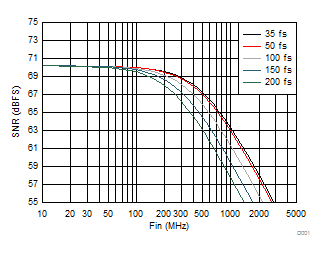Figure 45. SNR vs Input Frequency and External Clock Jitter